The nucleon induced fission cross section and the fragment distribution are calculated using the Quantum Molecular Dynamics (QMD) plus Statistical Decay Model (SDM) in the nucleon energy range 10 MeV to 10 GeV. The fission cross section is calculated for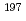Au,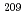Bi and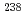U and compared with experiments. The calculation predicts fairly well the experimental values. The mass and charge distribution is calculated for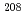Pb and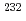Th at 1.6-GeV and 190-MeV proton incident energy, respectively.